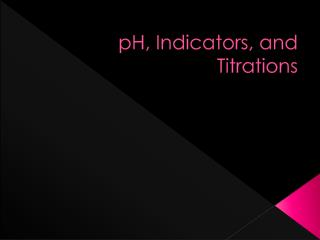# pH, Indicators, and Titrations - PowerPoint PPT PresentationDownload PresentationpH, Indicators, and Titrations

pH, Indicators, and Titrations
Download Presentation## pH, Indicators, and Titrations

- - - - - - - - - - - - - - - - - - - - - - - - - - - E N D - - - - - - - - - - - - - - - - - - - - - - - - - - -
##### Presentation Transcript

1. pH, Indicators, and Titrations

2. Chemistry Joke

3. Special thanks to Kate Pancho!

4. Definition of pH • pH is a number scale from 0 – 14 that represents the acidity or basicity of a solution. • 0 – 6 is acidic • 7 is neutral • 8 – 14 is basic

5. Definition of pH • pH is a measure of the hydrogen ion concentration of a solution • pH = - log [H+] • The lowercase p stands for negative log • [H+] represents the concentration of hydrogen ions in units of molarity (mol/L)

6. Definition of pOH • pOH is also a number scale that represents acidity or basicity of a solution. • It is the opposite of the pH scale • pOH is a measure of the hydroxide ion concentration of a solution. • pOH = - log [OH-]

7. pH and pOH • pH + pOH = 14 • [H+] [OH-] = 1 x 10-14 M • If [H+] increases, [OH-] must decrease.

8. Calculating pH and pOH • If [H+] is 10-5 M, the pH is 5. • Is this acidic, basic, or neutral? • If the pH is 5, what is the pOH? • pH + pOH = 14, so the pOH = 9. • If [H+] is 10-7 M, what is the pH? • Is this acidic, basic or neutral? • If the pH is 7, what is the pOH?

9. Calculating pH and pOH • If [OH-] = 10-5 M, what is the pOH? • If [OH-] = 10-6 M, what is the pH? • The pOH = 6, so the pH = 8 • If the hydrogen ion concentration is 1 x 10-4 M, is the solution acidic, basic, or neutral? • pH = 4, so acidic • If the hydroxide ion concentration is 1 x 10-5 M, is the solution acidic, basic, or neutral? • pOH = 5; pH = 9, so the solution is basic

10. Calculating [H+] and [OH-] • Remember that 10a x 10b = 10(a+b) • If [OH-] = 10-6 M, what is the [H+]? • [H+] [OH-] = 1 x 10-14 M • [H+] = 10-8 M • If [H+] = 10-9 M, what is the [OH-]? • [OH-] = 10-5 M • Fill in the chart on your notesheet.

11. Indicators • An indicator is a compound that changes color within a specific pH range. • Some common indicators: • Phenolphthalein—colorless to bright pink at a pH range of 8 – 10. • Alizarin yellow—yellow to lilac at a pH range of 10 – 12 • Bromphenol blue—yellow to blue at a pH range of 3 - 4

12. Indicator Demo

13. Titrations • Titration is a method used to determine the concentration of [H+] or [OH-]. • Procedure: • 1. Measure a volume of acidic solution of unknown concentration. • 2. Add an indicator to the solution. • 3. Add, drop by drop, a measured volume of base of known concentration just until the indicator turns color.

14. Titrations • The solution of known concentration is called the standard solution. • The point where the indicator changes color is called the end point. • Once the titration is complete, the unknown molarity of the acid can be calculated. • MA x VA = MB x VB • Molarity of acid x volume of the acid is equal to the molarity of the base x the volume of the base.

15. Titration Demo

16. Chemistry Joke Q: What is this? A: A fish out of water!!